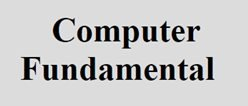# Algorithm and Flowchart

## Algorithm

Algorithm is a step by step description of how to arrive at a solution of any given problem.

Or you can say that, an algorithm of any program is the step by step solution description of the given program or problem.

In order to qualify as an algorithm, a sequence of instruction must posses these characteristics:

• Each instruction written should be precise and unambiguous
• Each instruction should be executed at a finite time
• None of any instruction should be repeated infinitely
• After executing all the given instruction, the desired result must be obtained

## Algorithm Example

Now let's take an example of algorithm, that how we can write an algorithm of a given problem.

#### Write an algorithm to find sum of first N numbers

Here we have to write an algorithm or the step by step solution description of finding sum of first N numbers.

```1. Read the value of n
2. Put i=1, sum=0
3. if(i>n) go to step 7
4. Update s = s + i
5. Update i = i+1
6. go to step 3
7. Print the value of s
8. Stop```

As you can see from the above algorithm written in 8 steps, helps you to make a program of finding sum of first N numbers.

#### Write an algorithm to find smallest and largest number from given list

Here we have to write an algorithm that will helps in finding the smallest and largest number from the given list of numbers.

```1. Read list of all numbers
2. Assign the first number as largest number
3. Assign the first number as smallest number
4. Repeat step 5, 6 and 7 as long as the largest and smallest number are there
5. Read number and check for largest and smallest with comparision
6. If the number is greater than largest then the current number is the largest.
7.If the number is lesser than smallest then the current number is the smallest.
8. Print Largest Number
9. Print Smallest Number
10. Stop```

Now you can easily apply all the above given steps to make your program that will find the largest and smallest number from the given list of numbers.

## Flow Chart

Flow chart is the pictorial representation of any given task or problem or program.

### Types of Flow Chart

There are two types of flow charts, given here with its short description:

1. System Flow Charts - These flowcharts describe the logical flow of the process, actually the sequence of events in business that happens before something is achieved
2. Program Flow Charts - This is a flowchart of a single program in high level language. This type of flow charts have the file names used for input, output, update of the files accessed and the names of the reports that might be created after the program run. It means, program flow charts contains each and every single details of the program

Here are some of the main and primary advantages of using the flow charts before applying any solution to a program:

1. Effective Analysis - Using flow chart, any problem can be analyzed in more effective way
2. Communication - Flowcharts are much better way of communicating any logic of a system to all concerned
3. Efficient Coding - Every flowcharts acts as a guide or blueprint during the systems analysis and program development phase
4. Proper Debugging - The flowcharts helps in debugging process
5. Efficient Program Maintenance - The maintenance of operating program becomes very easy using flowcharts.
6. Proper Documentation - Program flowcharts serve as a good program documentation, which is needed for various purposes

Here are the list of some primary or main disadvantages of using flowcharts for any given problem:

1. Complex Logic - Sometime, the given program logic is quit complicated. In that case, it becomes very difficult task to design or draw a flowchart for that problem or program. In that case, algorithm helps a lot
2. Alterations and Modifications - If we want to alter or change any logic of the program in the flowchart, then we have to re-draw the whole flowchart which is very irritating.

### Basic Symbols used in Flow Chart

The American National Standard Institute (ANSI) has standardized these given basic flow chart symbols.

#### Terminal

Terminal symbol is used to indicate the start and end of the program. Here is the symbol of terminal: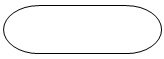#### Input/Output

A parallelogram is used to represent an input and output representation or operation. Here is the demo symbol of a input/output used in flowchart: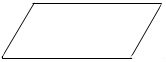#### Processing Symbol

To represent any processing operation in flowchart, we have to use the rectangle symbol. Here is the demo symbol of rectangle used in drawing flowchart to use storage or arithmetic operation: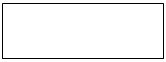#### Decision Symbol

To indicate the step of decision making statement, we have to use diamond shaped box. Here is the demo symbol of diamond shaped box: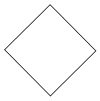#### Flow of Lines

Flow of lines indicates the path of logic flow in a program. Between each boxes used in flowchart, we have to use lines to indicate where the program flow goes. Here is the demo symbol of lines flow: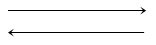#### Connector Symbol

We have to use circle to join different parts of a flowcharts. Here is the demo symbol of circle used in flowchart: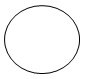### Flowchart Example

Now let's take an example of flowchart to understand it in a clear way that how to use all the symbols given above and draw pictorial representation that tells the solution of any program or problem.

#### Draw flowchart to find largest of three numbers

Here the figure given below shows the flowchart that helps in finding the largest of three numbers:#### Draw flowchart to find sum of first N numbers

Below is another flowchart of finding the sum of first N natural numbers:Tools
Calculator